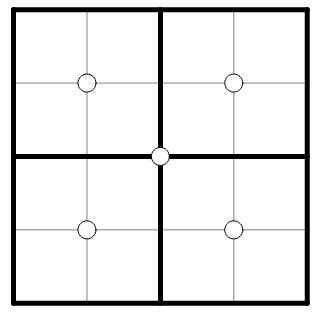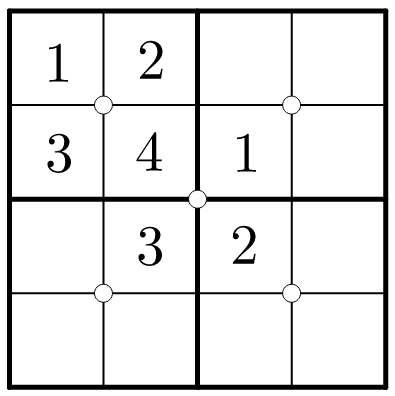# Sum SudokuHere are the rules of sum Sudoku:

• Each and every column, row, and $2\times2$ box contains distinct numbers from 1 to 4.
• The white circle marked at four adjacent cells indicate that the sum of upper-left and bottom-right cells is the sum of upper-right and bottom-left cells.

What can we conclude about the circle sums in the puzzle?


Example:
The setup in the top-left box is valid since $2 + 3 = 1 + 4 = 5$. However, the four cell values at the center are invalid since $4 + 2 \neq 3 + 1$.×